Latest Banking jobs   »   Quantitative Aptitude Quiz for IBPS 2020...

Quantitative Aptitude Quiz for IBPS 2020 Mains Exams- 3rd December

Directions (1-5): Each of the following question is followed by two quantities I, and II. You have to determine the value of the quantities using the information provided and compare the quantities to answer as per the instruction set provided below.
(a) Quantity I>Quantity II
(b) Quantity I≥Quantity II
(c) Quantity I<Quantity II
(d) Quantity I≤Quantity II
(e) Quantity I=Quantity II or no relation

Q1. What is the number?
Quantity I: 40% of number is 12.
Quantity II: 50% of 20 is number.

Q2. Find the time taken to cover 30 km.
Quantity I: the speed of bike is 20 kmph.
Quantity II: a car covers 20 km in 30 minutes.

Q3. Find the present population of town.
Quantity I: the population increases every year by 10% and last year it was 10000.
Quantity II: male population is 30% more than female population which is 5000.(population= male+ female)

Q4. Quantity I: marks obtained in Maths is 90 which is 10% less than marks obtained in Science. What is average marks in both subjects?
Quantity II: 95

Q5. Quantity I: Prem saves Rs. 5000 per month from annual salary of Rs. 180000. Find expenditure per month.
Quantity II: Rahul spends at least 60% of his income and saves Rs. 4000 per month. What can be his monthly salary?

Directions (6-10): In the given questions, two quantities are given, one as ‘Quantity I’ and another as ‘Quantity II’. You have to determine relationship between two quantities and choose the appropriate option:

Q6.  Ratio of age of Anurag and Bhumi is 6 : 7 and Dharmendra : Ekta is 9 : 5. Ratio of average age of Anurag, Bhumi, Dharmendra& Ekta to age of Chiru is 9 : 10
Quantity I- If ratio of age of Bhumi and Dharmendra is 7 : 9 and age of Chiru is 6 years less than age of Dharmendra, then age of Chiru.
Quantity II – If ratio of age of Bhumi and Dharmendra is 7 : 9 and age of Chiru is 6 years less than age of Dharmendra, Average age of Anurag& Dharmendra.
(a) Quantity I > Quantity II
(b) Quantity I < Quantity II
(c) Quantity I ≥ Quantity II
(d) Quantity I ≤ Quantity II
(e) Quantity I = Quantity II or no relation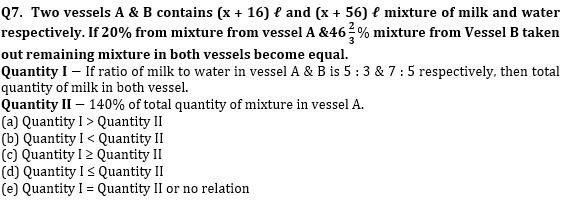Q8.  Radius of cone is 4 cm less than side of square of which area is 324 cm2. Height of cone is 5 cm more than radius of circle, whose circumference is 44 cm.
Quantity I –Volume of cone.
Quantity II –2488 cm3
(a) Quantity I > Quantity II
(b) Quantity I < Quantity II
(c) Quantity I ≥ Quantity II
(d) Quantity I ≤ Quantity II
(e) Quantity I = Quantity II or no relation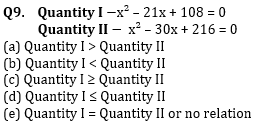Q10.  240 meters & 210 meters long trains running in opposite direction cross each other in 6 sec. Ratio between speed of longer train and smaller train is 7 : 8 and faster train cross a platform in 9 sec.
Quantity I – Time taken (in sec) by slower train to cross a bridge, which is 60 meters longer than platform.
Quantity II –16 sec
(a) Quantity I > Quantity II
(b) Quantity I < Quantity II
(c) Quantity I ≥ Quantity II
(d) Quantity I ≤ Quantity II
(e) Quantity I = Quantity II or no relation

Directions (11-15): Each of the following question is followed by two quantities I, and II. You have to determine the value of the quantities using the information provided and accordingly compare the quantities. Mark your answer as per the instruction set provided below.
(a) Quantity I>Quantity II
(b) Quantity I≥Quantity II
(c) Quantity I<Quantity II
(d) Quantity I≤Quantity II
(e) Quantity I=Quantity II or no relation

Q11. Quantity I: Time taken by Prabhas to cover a distance of 200 km (without stoppage) by car through which he covers a distance of 100 km in 1 hour when he stops to get fuel for 10 minutes.
Quantity II: Time taken by Nagarjuna to travel 20 km downstream if speed of boat and speed of stream be 7 kmph & 3 kmph respectively.

Q12. There are 3 red and 5 blue balls in the urn.
Quantity I: the probability of drawing 1 red ball and 2 blue balls.
Quantity II: the probability of drawing 2 red balls and 1 blue ball.

Q13. In how many ways can 4 boys or 5 girls be selected?
Quantity I: there are 20 persons (boys and girls only) in the group out of which 12 are boys.
Quantity II: the group comprises 10 boys and 10 girls.

Q14. Quantity I: The length & breadth of a rectangle of perimeter 48 cm are in ratio 5:3. Find Area?
Quantity II: 135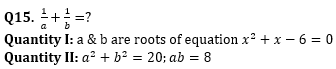Practice More Questions of Quantitative Aptitude for Competitive Exams:

Solutions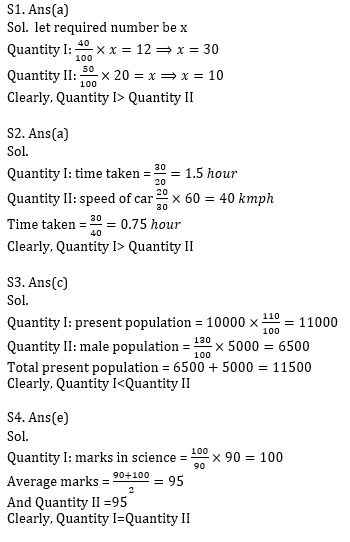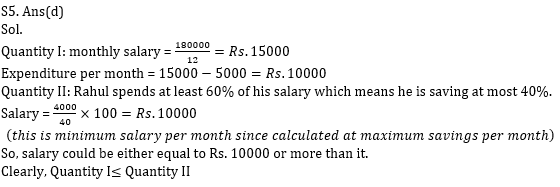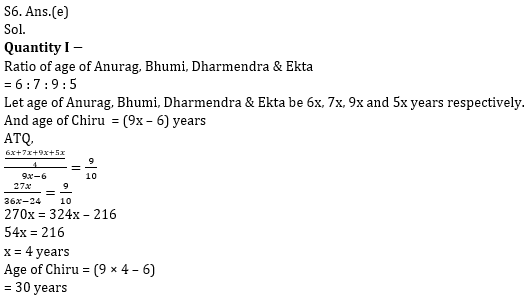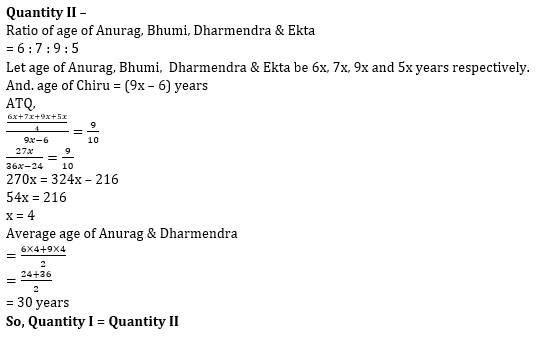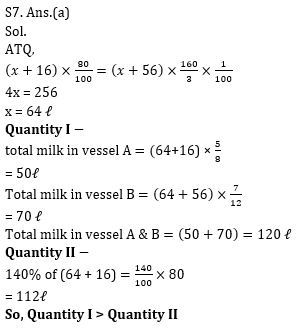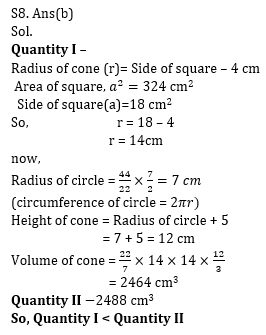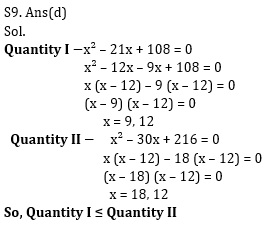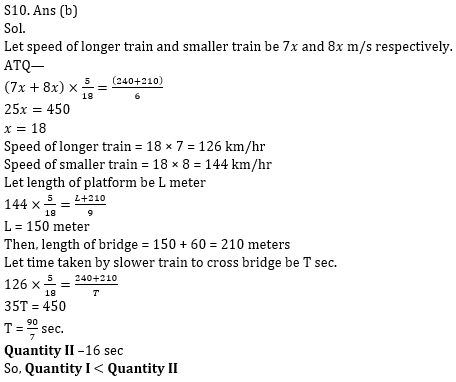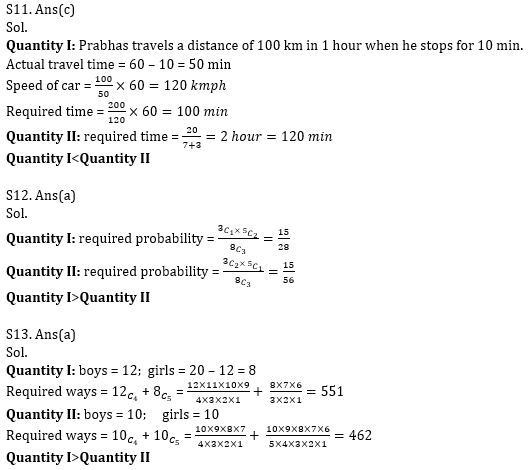Practice with Crash Course and Online Test Series for IBPS Clerk Prelims:×

Thank You, Your details have been submitted we will get back to you.Join India's largest learning destination

What You Will get ?

•Job Alerts
•Daily Quizzes
•Subject-Wise Quizzes
•Current Affairs
•Previous year question papers
•Doubt Solving session

ORJoin India's largest learning destination

What You Will get ?

•Job Alerts
•Daily Quizzes
•Subject-Wise Quizzes
•Current Affairs
•Previous year question papers
•Doubt Solving session

ORJoin India's largest learning destination

What You Will get ?

•Job Alerts
•Daily Quizzes
•Subject-Wise Quizzes
•Current Affairs
•Previous year question papers
•Doubt Solving session

Enter the email address associated with your account, and we'll email you an OTP to verify it's you.Join India's largest learning destination

What You Will get ?

•Job Alerts
•Daily Quizzes
•Subject-Wise Quizzes
•Current Affairs
•Previous year question papers
•Doubt Solving session

Enter OTP

Please enter the OTP sent to
/6

Did not recive OTP?

Resend in 60sJoin India's largest learning destination

What You Will get ?

•Job Alerts
•Daily Quizzes
•Subject-Wise Quizzes
•Current Affairs
•Previous year question papers
•Doubt Solving sessionJoin India's largest learning destination

What You Will get ?

•Job Alerts
•Daily Quizzes
•Subject-Wise Quizzes
•Current Affairs
•Previous year question papers
•Doubt Solving session

Almost there

+91Join India's largest learning destination

What You Will get ?

•Job Alerts
•Daily Quizzes
•Subject-Wise Quizzes
•Current Affairs
•Previous year question papers
•Doubt Solving session

Enter OTP

Please enter the OTP sent to Edit Number

Did not recive OTP?

Resend 60

By skipping this step you will not recieve any free content avalaible on adda247, also you will miss onto notification and job alerts

Are you sure you want to skip this step?

By skipping this step you will not recieve any free content avalaible on adda247, also you will miss onto notification and job alerts

Are you sure you want to skip this step?